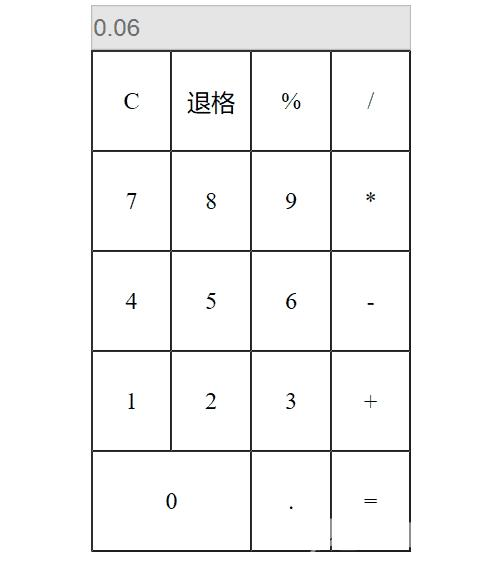﻿ JavaScript实现网页版简易计算器功能 - 自由资讯

# JavaScript实现网页版简易计算器功能```<!DOCTYPE html>
<html>
<meta charset="UTF-8">
<title>网页版的简易计算器</title>
<!--使用js完成,之后可用:jquery-->
<!-
-->
<style>
/*设置input的宽度和高度*/
input{
width: 396px;
height: 50px;
font-size: 30px;
}

/*表格的行文本左右居中*/
tr{
/*text-align*/
text-align: center;
}
/*设置单元格的内容宽度和高度以及字体大小*/
td{
width: 100px;
height: 119px;
font-size:30px;
}
/*伪类选择器:hover*/
td:hover{
/*指定背景色*/
background-color: greenyellow;
/*cursor:pointer
* 鼠标经过的时候,变成小手
*/
cursor:pointer ;
}
</style>
<body>
<!--
cellspacing:单元边沿和单元格之间的空间
-->
<!--在input上面写一个div: 指定这个当前系统时间-->
<!--禁用文本框的输入功能:disabled:disabled-->
<center><input type="text" value="0" id="show" disabled="disabled" /></center>
<table border="1px" cellspacing="0" cellpadding="2" align="center" width="400px"
height="600px">
<tr>
<td id="clear">C</td>
<td id="del">退格</td>
<td>%</td>
<td class="operator">/</td>
</tr>
<tr>
<td class="num">7</td>
<td class="num">8</td>
<td class="num">9</td>
<td class="operator">*</td>
</tr>
<tr>
<td class="num">4</td>
<td class="num">5</td>
<td class="num">6</td>
<td class="operator">-</td>
</tr>
<tr>
<td class="num">1</td>
<td class="num">2</td>
<td class="num">3</td>
<td class="operator">+</td>
</tr>
<tr>
<td colspan="2" class="num">0</td>
<td class="num">.</td>
<td id="result">=</td>
</tr>
</table>
</body>
<script>
/**
* -
1.页面布局
要么使用div+css,要么table布局
table布局:5行4列的表格
div+css布局:大的div  嵌套两个字div(输入框的和键盘)
2.逻辑处理
2.1 将数字键和小数点看成一类:clas="num"
2.2 将运算符看成一类:class="operator"
2.3 将清除键,退格键,=(等号),显示框,单独设置id属性
2.4 获取显示框,清除键,=号,推格键所在的标签对象
2.5 获取数字键所在的对象并设置点击事件
2.6 获取运算符所在的对象并设置点击事件
*/
//获取显示框所在的标签对象
var showResult = document.getElementById("show") ;
//获取清除键所在的标签对象
var clear = document.getElementById("clear") ;
//获取退格键所在的标签对象
var del = document.getElementById("del") ;
//获取等号所在的对象
var result = document.getElementById("result") ;

//定义三个变量,分别保存第一个数,第二个数以及运算符
//第一个数
var numValue1 = "" ;
//第二个数
var numValue2 = "" ;
//操作符
var oper ="" ;

//获取数字键,包含小数点,并设置点击事件
var nums = document.getElementsByClassName("num") ;
//遍历数字,分别设置点击事件
for(var i = 0 ; i< nums.length ; i++){
//设置点击事件:使用匿名函数
nums[i].onclick = function(){

//小数点的问题：只能出现一个：
/**
* 如果这个this.innerTest==.
*         //小数点只能出现一个
* 否则：
*         不是小数点，就属于整数运算
*         numValue1 += this.innerText;
showResult.value = numValue1*1 ; //去掉首尾无效0
*/

//测试
//将点击的数字显示到文本框上
//将nums[i]--->看成一个this:代表当前数字键对象的内存地址值
//var text = this.innerText ; //普通文本
//测试
//改变显示框对象的value属性
//showResult.value = text;

//问题1:目前:用户输入第一个数只能是一位数的
//将数字内容赋值给第一个数,然后将第一个数展示到文本框上
//        numValue1 = this.innerText ;
numValue1 += this.innerText;
//        showResult.value = numValue1*1 ; //去掉首尾无效0
showResult.value = numValue1 ; //去掉首尾无效0

//问题2:小数点的问题(小数点只能出现一个)

}
}

//获取运算符所在的对象并设置点击事件
var operators = document.getElementsByClassName("operator") ;
//遍历操作符,设置点击事件
for(var i = 0 ; i < operators.length ; i++){
//设置点击
operators[i].onclick = function(){

/**
* 判断numValue2的值是否为空：
*         如果是numValue2==""
*         如果numValue2不是空字符串
*             用户可能点击等号或者运算符
*/
if(numValue2==""){
numValue2 = numValue1 ;
numValue1 = "" ;
//保存操作符
oper = this.innerText ;
}else{
//用户要么点击=号要么进行四则运算
//问题：应该将计算的结果赋值numValue2,清空numvalue1

if(numValue1!=""){
resultFn() ;
}

//保留操作符
oper = this.innerText ;
}

//测试
//做运算 :思考如何做运算,最终处理
//将第二个数赋值第一个数,然后将第一个数清空,等待接收用户下一次输入的数据
/*    numValue2 = numValue1 ;
numValue1 = "" ;
//保存操作符
oper = this.innerText ;*/
}
}

//关于其他的操作:清除键
//设置清除键的点击事件
clear.onclick = function(){

//将第一个数,第二个数以及运算符都情况掉
//显示框显示默认值0
numValue1 = "" ;
numValue2 = "" ;
oper = "" ;
showResult.value = "0" ;
}

//删除键的逻辑,设置点击事件
del.onclick = function(){

//可以用到String :substring()截取功能
if(numValue1.length!=1){
//进行截取:从0索引开始截取到当前numValue1.length-1处的位置结束,将其值
//赋值给numValue1
//场景:删除剩余一个数字即可
numValue1 = numValue1.substring(0,numValue1.length-1) ;
//将numValue1显示文本框上
//    showResult.value = numValue1 *1 ; //去掉首位无效0
showResult.value = numValue1 ; //
}
//    else{
//        //自己处理
//将第一个数,第二个数,运算符清空掉
//    }
}

//给等号添加点击事件,做四则运算

result.onclick = function(){

//抽取出一个方法
resultFn() ;
}

//具体做四则运算的方法
function resultFn(){

//定义运算的两个数据: one ,two
//数据类型转换
var one = new Number(numValue2)    ;//实际是第二个数
var two = new Number(numValue1) ;//实际第一个数
//保存计算结果
var r = null ;
//根据运算符进行判断，switch语句
switch(oper){
case "+":
r = one + two ;
break ;
case "-":
r = one - two ;
break;
case "*":
r = one * two ;
break ;
case "/":
//除数不能为0
//判断
/*    if(two==0){
//将数据情况掉
//并且弹框提示；除数不能为0

r = 0
}else{
one /two ;
}*/
r = one /two ;

break ;
}

//将计算结果展示到显示框上
//    showResult.value = r ;

//将计算的结果进行保留小数点的有戏位
//    numValue2 = r ;
numValue2 = new Number(r).toFixed(6) ;
numValue1 = "" ; //numValue1清空了
showResult.value = numValue2*1; // numValue2*1

//问题1 :1.2 * 3 = 3.599999996
//问题2：去掉首尾无效0    ：parseFloat()
//问题3：在四则元素：判断用户如果是点击等号或者点击继续运算，每一次都将第一个数清空掉了，
//判断第一个数是否为空

//问题4：小数点问题：小数点只能出现一个

//四则运算的问题：
/**
* 用户输入两个数据目前是直接处理结果，那么如何判断用户是要点击=号还是继续进行四则运算？
*     在运算符点击监听事件中去坐
* 判断numValue2的值是否为空：
*         如果是numValue2==""
*         如果numValue2不是空字符串
*             用户可能点击等号或者运算符
*
*/

}

</script>
</html>```

﻿